### Home > CALC > Chapter 2 > Lesson 2.2.4 > Problem2-95

2-95.
1. Inscribed rectangles are below a curve. Circumscribed rectangles are above a curve. Homework Help ✎
For the function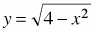, complete the following problems.

1. Calculate the area from −2 ≤ x ≤ 2 using four inscribed rectangles

2. Calculate the area from −2 ≤ x ≤ 2 using four circumscribed rectangles.

3. Estimate the actual area using your answers to parts (a) and (b).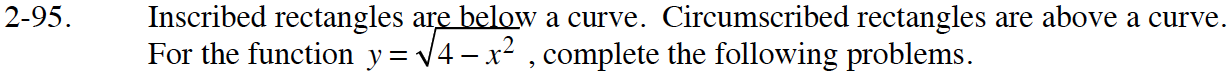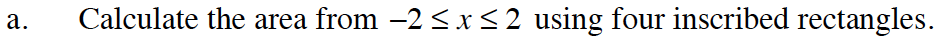Some of the rectangles will be left-endpoint and others will be right-endpoint.

Inscribed means that only one point of the inscribed shape is touching the function. The rest of the points lie within the function.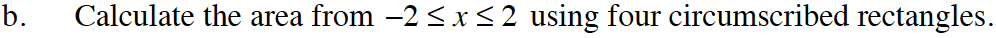Circumscribed means the shape you are drawing must entirely contain the function. The function is inside the shape.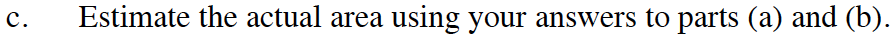What shape is this? Use geometry.

Use the eTool below to view the graph of the shapes.
Click the link to the right to view the full version of the eTool: Calc 2-95 HW eTool